# AP Calculus AB Practice Test 54

### Test Information8 questions24 minutes

Calculator Allowed

1. The position function of a moving particle is s(t) = 5 + 4t&- t2for 0 ≤ t≤ 10 where sis in meters and tis measured in seconds. What is the maximum speed in m/sec of the particle on the interval 0 ≤ t≤ 10?

2. How many points of inflection does the graph of y= cos (x2) have on the interval (0, π)?

3. Let fbe a continuous function on [4, 10] and have selected values as shown below: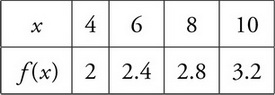Using three right endpoint rectangles of equal length, what is the approximate value of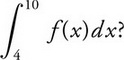4. Given a differentiable function fwith f(&- 1) = 2 and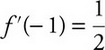. Using a tangent line to the graph of fat x= &- 1 find an approximate value of f(&- 1.1)?

5. If area under the curve of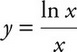is 0.66 from x= 1 to x= b, where b> 1, then the value of bis approximately,

6. The base of a solid is a region enclosed by the circle x2+ y2= 4. What is the approximate volume of the solid if the cross sections of the solid perpendicular to the x-axis are semicircles?

7. The temperature of a cup of coffee is dropping at the rate of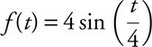degrees for 0 ≤ t≤ 5, where fis measured in Fahrenheit and tin minutes. If initially, the coffee is 95°F, find its temperature to the nearest degree Fahrenheit 5 minutes later.

8. The graphs of f′, g′, p′, and q′ are shown below. Which of the functions f, g, p, or qhave a relative minimum on (a, b)?